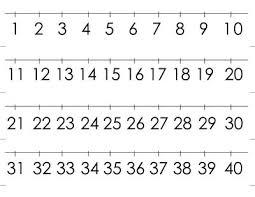# Increase 5366

I think the number. If I increase it by 25%, I get the number 100. What number do I think?

x =  80

### Step-by-step explanation:

x + 25/100 x = 100

x + 25/100·x = 100

125x = 10000

x = 10000/125 = 80

x = 80

Our simple equation calculator calculates it.Did you find an error or inaccuracy? Feel free to write us. Thank you!

Tips for related online calculators